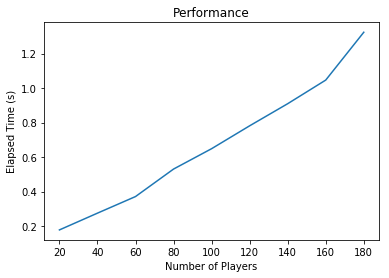# Efficiency and Performance¶

:

%matplotlib inline
import time
import pymarket as pm
import numpy as np
import matplotlib.pyplot as plt


## Create a set of markets with varying number of participants¶

:

markets = []
range_players = np.arange(20, 200, 20)
M = len(range_players)

for i in range_players:
bids = pm.datasets.generate(i, i, 2, 1)
mar = pm.Market()
for b in bids:
mar.accept_bid(*b)
markets.append(mar)


## Run the diferent markets¶

:

elapsed = np.zeros(M)
for i in range(M):
mar = markets[i]
start = time.time()
mar.run('huang')
stop = time.time()
elapsed[i] = stop - start

:

fig, ax = plt.subplots()
ax.plot(range_players, elapsed)
_ = ax.set_xlabel('Number of Players')
_ = ax.set_ylabel('Elapsed Time (s)')
_ = ax.set_title('Performance')## Obtains the statistics (optimization problems have to be solved)¶

:

traded = np.zeros(M)
welfare = np.zeros(M)
stats_time = np.zeros(M)

limit = M
for i in range(limit):
mar = markets[i]
start = time.time()
stats = mar.statistics()
stop = time.time()
stats_time[i] = stop - start
welfare[i] = stats['percentage_welfare']


## Plots the results¶

:

fig, ax = plt.subplots(1, 3, figsize=(18, 6))

ax.plot(range_players[:limit], stats_time[:limit])
ax.set_ylabel('Elapsed Time (s)')

ax.plot(range_players[:limit], welfare[:limit])
ax.set_ylabel(' % Total welfare')[ ]: